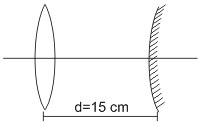#### In an experiment a convex lens of focal length 15 cm is placed coaxially on an optical bench in front of a convex mirror at a distance of 5 cm from it.  It is found that an object and its image coincide, if the object is placed at a distance of 20 cm from the lens.  The focal length of the convex mirror is : Option 1)      27.5 cm Option 2)  20.0 cm Option 3)  25.0 cm Option 4) 30.5 cmAs we learnt in

Mirror Formula -- whereinObject distance from pole of mirror.Image distance from pole of mirror.focal length of the mirror.Given focal length of lents (f) = 1.5 cm

Object distance u = - 20 cm ,  f = 15 cm

We know thatv = 60 cm

The image 1 gets formed at 60 cm to ghe right of the lens and it will be inverted.

Therefore, Distance between lens and mirror will be

d = image distance(v) - radius of curvature of convex mirror

s = 60 - 2f2f = 60 - 5(convex mirror)

Correct option is 1.

Option 1)

27.5 cm

This the correct option.

Option 2)

20.0 cm

This is an incorrect option.

Option 3)

25.0 cm

This is an incorrect option.

Option 4)

30.5 cm

This is an incorrect option.

##### Posted by